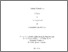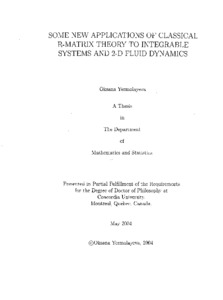Title:

Some new applications of classical r-matrix theory to integrable systems and 2-D fluid dynamics

Yermolayeva, Oksana (2004) Some new applications of classical r-matrix theory to integrable systems and 2-D fluid dynamics. PhD thesis, Concordia University.Preview
Text (application/pdf)
NQ96945.pdf - Accepted Version
5MB

Abstract

This thesis combines three different problems related to integrable systems and 2D fluid dynamics; united through the R-matrix approach to integrable systems. The first part is devoted to the Krall-Sheffer theory of two-dimensional classical orthogonal polynomials. This problem is deeply connected with the classification of second order linear differential operators in 2D having polynomial eigenvectors with certain degeneracies of eigenvalues. The original classification problem is related to the theory of symplectic reductions of Hamiltonian systems. Using the R-matrix theory on loop algebras, the reduction scheme sheds new light on the superintegrability of Krall-Sheffer operators. It is also used to deduce explicit results on separation of coordinates and commuting invariants in both the classical and quantum settings. In the second part of the thesis we study Poisson structures on spaces of rational maps. Such spaces have natural Poisson structures discovered by Atiyah and Hitchin. The approach developed here relates those to the classical R -matrix scheme and further generalizes the Atiyah-Hitchin construction to spaces of meromorphic functions in different geometries using the quadratic r-matrix construction of Sklyanin. More precisely, any classical R -matrix may be viewed as a special linear automorphism of a Lie algebra. In the Sklyanin theory, the r-matrix defines simultaneously a quadratic Poisson structure on a Poisson-Lie group and a linear one on the corresponding dual space to the Lie algebra. The quadratic structures appeared first as Poisson brackets between elements of the scattering matrix in the case of rapidly decreasing boundary conditions. The Atiyah-Hitchin structure is also defined as brackets on a space of scattering matrices for solutions of Bogomolny equations governing interactions of monopoles in nonabehan gauge theories. Using this analogy, we obtain the desired generalizations. The third project relates to the problem of Laplacian Growth (LG) in two-dimensional viscous fluid dynamics. It describes the dynamics of the boundaries in various processes (e.g. propagation of oil-water interfaces, crystal growth etc), and is of great practical importance. Recently established connections with integrable systems has brought the problem into the domain of modern mathematical physics. In this thesis, the Hamiltonian nature of rational conformal maps describing so-called "multi-finger" solutions of the LG problem is investigated. (Abstract shortened by UMI.)

Divisions: Concordia University > Faculty of Arts and Science > Mathematics and Statistics Thesis (PhD) Yermolayeva, Oksana 1 v. (various pagings) : ill. ; 29 cm. Concordia University Ph. D. Mathematics 2004 Harnad, J 8051 Concordia University Library 18 Aug 2011 18:14 18 Jan 2018 17:32 http://clues.concordia.ca/search/c?SEARC...
All items in Spectrum are protected by copyright, with all rights reserved. The use of items is governed by Spectrum's terms of access.

Repository Staff Only: item control pageResearch related to the current document (at the CORE website)
Back to top